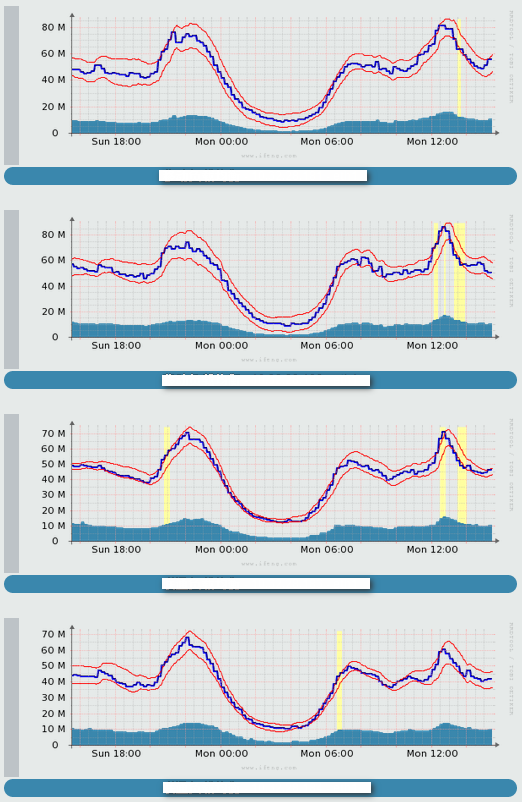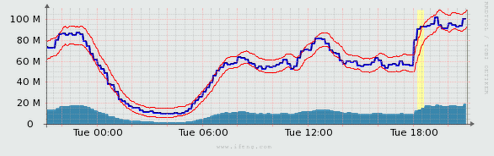# 流量异常行为检查算法

#### 1. 基础 - 异常行为检查算法

• 拥有一个随时间序列变化，预测未来值的算法
• 在真实的观测值和预测值之前计算出一个差值
• 最后，建立一套机制用于判断所观测到的值是否异常，什么时候出现的异常

#### 2. 进阶 - 利用rrdtool进行数据收集

``````
rrdtool create monitor.rrd –s 300 \
DS:ifOutOctets:COUNTER:1800:0:4294967295 \
RRA:AVERAGE:0.5:1:2016 \
RRA:HWPREDICT:1440:0.1:0.0035:288

``````

``````
RRA:HWPREDICT:<array length>:<alpha>:<beta>:<period>

Where:
<array length> is the number of predictions to store before wrap-around; this number must be longer than the seasonal period. This value will also be the RRA row count for DEVPREDICT RRA.
<alpha> is the intercept adaptation parameter, which must fall between 0 and 1. A larger value means the intercept adapts faster. The same value will be also be used for gamma.
<beta> is the slope adaptation parameter, again between 0 and 1.
<period> is the number of primary data points in the seasonal period. This value will be the RRA row counts for the SEASONAL and DEVSEASONAL RRAs.

Using this option creates the FAILURES RRA with the default window length of 9 and a default threshold value of 7. The default length of the FAILURES RRA is one period.

``````

``````
rrdtool graph example.gif \
DEF:obs=monitor.rrd:ifOutOctets:AVERAGE \
DEF:pred=monitor.rrd:ifOutOctets:HWPREDICT \
DEF:dev=monitor.rrd:ifOutOctets:DEVPREDICT \
DEF:fail=monitor.rrd:ifOutOctets:FAILURES \
TICK:fail#ffffa0:1.0:"Failures Average bits out" \
CDEF:scaledobs=obs,8,* \
CDEF:upper=pred,dev,2,*,+ \
CDEF:lower=pred,dev,2,*,- \
CDEF:scaledupper=upper,8,* \
CDEF:scaledlower=lower,8,* \
LINE2:scaledobs#0000ff:"Average bits out" \
LINE1:scaledupper#ff0000:"Upper Bound Average bits out" \
LINE1:scaledlower#ff0000:"Lower Bound Average bits out"

``````

#### 3. 成果 - 展示图形